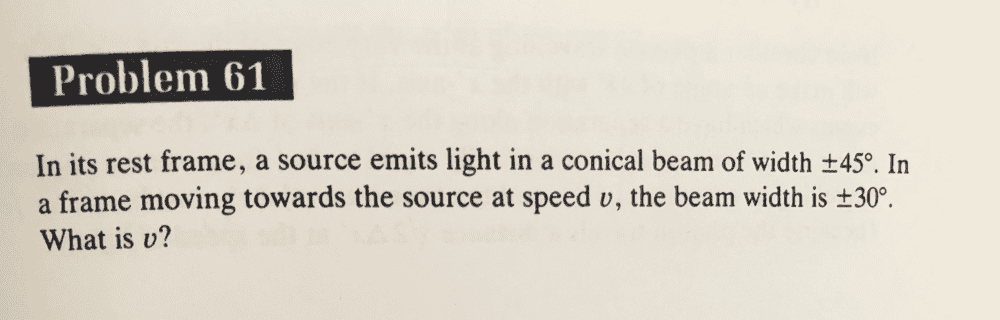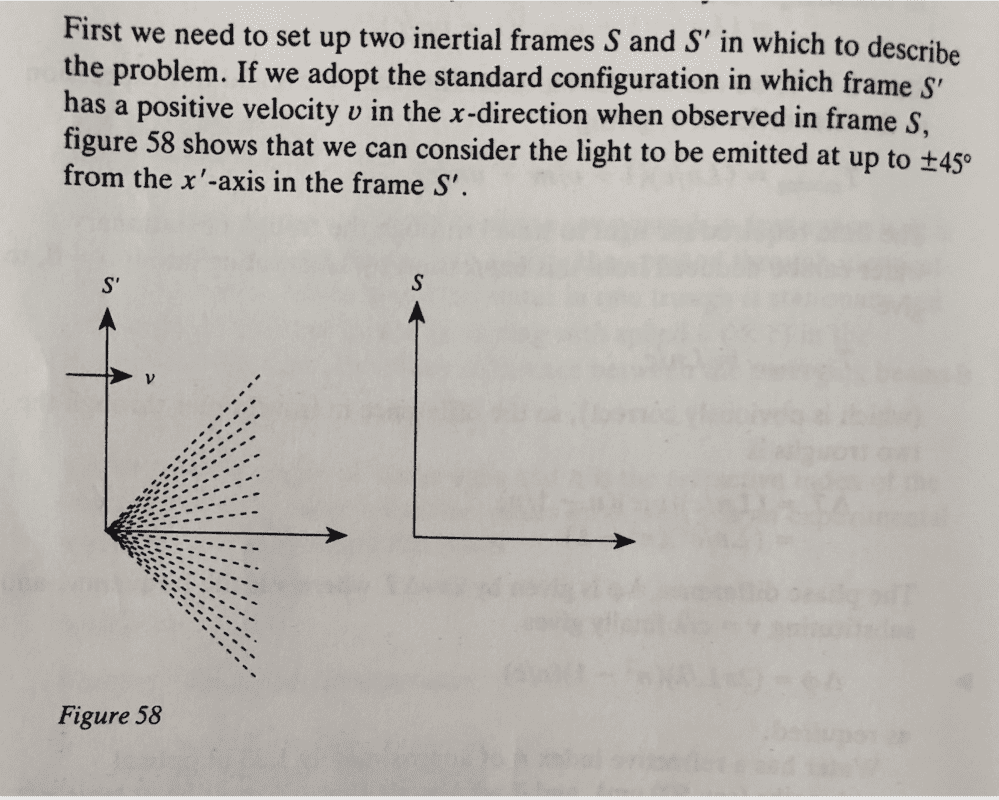# Velocity addition formula in X and Y axes (Relativity)

phantomvommand
Homework Statement:
Relevant Equations:
Velocity addition formula (in X and Y axes)
The problem:Visualising the problem (My question is with regards to this):Why is the above set-up correct? In the above diagram, S would be moving at velocity -v relative to S', instead of v. Is this because the question says "speed v", and so we can set the direction as such? Why would the opposing direction of v be incorrect?

Last edited:

mitochan
The rest frame of light source is S'. I think the better way to illustrate would be :

This is an illustration in S. The light cone angle be not 45 degree as drawn in Fig.58 but 30 degree.

or

This is an illustration in S'. The arrow of v on S' axis drawn in Fig.58 be deleted but arrow of opposite direction v should be drawn on S axis.

phantomvommand
The rest frame of light source is S'. I think the better way to illustrate would be :

This is an illustration in S. The light cone angle be not 45 degree as drawn in Fig.58 but 30 degree.

or

This is an illustration in S'. The allow of v on S' axis drawn in Fig.58 be deleted but allow of opposite direction v should be drawn on S axis.
Yes, The rest frame of light is S'. But S is moving at speed v with respect to S'. In the diagram, it shows S moving at velocity -v with respect to S', why is it not correct to show S moving at velocity v with respect to S'? (which is the same as S' moving at -v with respect to S)

mitochan
The diagram shows light source at rest in S' is approaching to S. it will come to origin of S and then surpass it and leave to infinite far away. In all the procedures light cone angle keeps 30 degree in S. So I think you do not have to keen on v or -v, approaching or leaving.

•phantomvommand
phantomvommand
The diagram shows S' is approaching to S. S' will superpose on S and then surpass it and leave to infinite far away.
Why not S' move away from S (move in the other direction)? Where in the question says this cannot be the case?

mitochan
Why don't you take v or -v as you like and solve the problem and then change the signature and solve it again?

•PeroK and phantomvommand
phantomvommand
Why don't you take v or -v as you like and solve the problem and then change the signature and solve it again?
I interpreted it as S' moving to the left, but the light moves to the right. Hence, I do not get the correct answer. So I only swapped the direction of v, but not the direction of the light. Why is this interpretation wrong?

mitochan
What is the wrong answer you got ?

Staff Emeritus
Homework Helper
Why is the above set-up correct? In the above diagram, S would be moving at velocity -v relative to S', instead of v. Is this because the question says "speed v", and so we can set the direction as such? Why would the opposing direction of v be incorrect?
Yes, it's because it says "speed" not "velocity".

Yes, The rest frame of light is S'. But S is moving at speed v with respect to S'. In the diagram, it shows S moving at velocity -v with respect to S', why is it not correct to show S moving at velocity v with respect to S'? (which is the same as S' moving at -v with respect to S)
You could, but you'd also have to change the relative positions of the origins of S and S' so that the observer at rest in S approaches the light source.

I interpreted it as S' moving to the left, but the light moves to the right. Hence, I do not get the correct answer. So I only swapped the direction of v, but not the direction of the light. Why is this interpretation wrong?
It's not clear what you did here. So the light is moving in both S and S'?

•phantomvommand
Gold Member
Yes, the problem expresses v as a speed, not a velocity. It is part of the problem to determine the direction of motion of the source in S since the angle gets larger * in the other direction.

* There are actually two solutions to the problem, but with the other solution, the angle of the cone is the same, but the light is on the outside and the interior of the cone is dark.

•phantomvommand